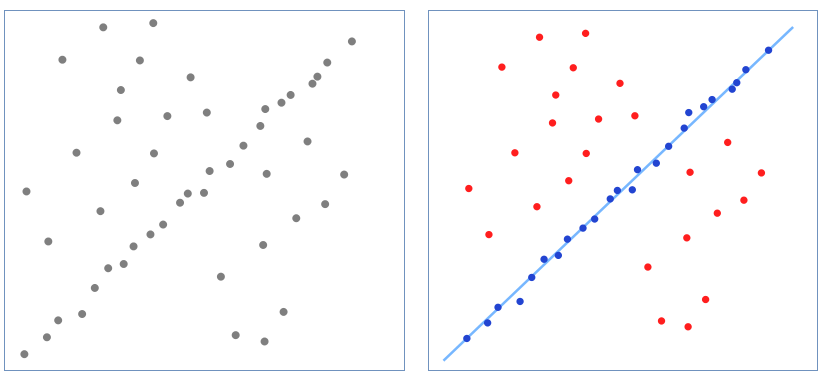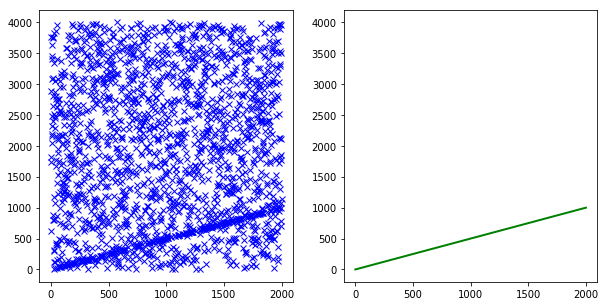RANSAC是Random Sample Consensus（随机采样一致）的缩写。

RANSAC是一种通用算法，可以与其他参数估计方法一起使用，当数据中存在大量离群（outilers，即异常值）时，估计拟合的内群（inliers）的数学模型参数

RANSAC是一种迭代方法，也是一个非确定性算法，在某种意义上说，它会产生一个在一定概率下合理的结果，而更多次的迭代会使这一概率增加。此RANSAC算法在1981年由Fischler和Bolles首次提出，随后产生了许多变体，并在机器视觉、模型估计（Model Fitting）等领域有着巨大影响。

for a better world without outliers...


## Introduction 介绍## Overview 概述

### Motivation

RANSAC就是解决这种问题一个算法，或者说一种思想，通过排除可能存在的错误数据，来估计模型参数。

### Assumptions

RANSAC假设数据由可以用模型解释的内群（inliers）组成，而离群（outliers）是完全不适合模型的错误数据。 因此，在训练模型时使用离群（outliers）会增加我们的最终预测误差，因为它们几乎不包含有关模型的信息。

RANSAC使用一小部分样本来估计模型参数，而不是使用所有的数据，虽然这是一个软性假设（soft assumption），然而对大多数情况都适用，例如拟合一个直线模型，两个数据样本就足够了。

### Method

RANSAC均匀随机地选择一个数据样本子集并用它来估计模型参数，然后选择数据集中，在生成模型的容错范围内的样本。

## Algorithm 算法

### Flow

RANSAC算法流程的一般描述如下所示：

Given:

data - a set of observed data points
model - a model that can be fitted to data points
n - the minimum number of data values required to fit the model
k - the maximum number of iterations allowed in the algorithm
t - a threshold value for determining when a data point fits a model
d - the number of close data values required to assert that a model fits well to data

Return:

bestfit - model parameters which best fit the data (or nil if no good model is found)

RUN

iterations = 0
bestfit = nil
besterr = something really large

while iterations < k
maybeinliers = n randomly selected values from data
maybemodel = model parameters fitted to maybeinliers
alsoinliers = empty set

for every point in data not in maybeinliers
if point fits maybemodel with an error smaller than t
add point to alsoinliers

if the number of elements in alsoinliers is > d
% this implies that we may have found a good model
% now test how good it is
bettermodel = model parameters fitted to all points in maybeinliers and alsoinliers
thiserr = a measure of how well model fits these points

if thiserr < besterr
bestfit = bettermodel
besterr = thiserr

increment iterations
return bestfit


The algorithm flow is referenced from Wikipedia (http://en.wikipedia.org/wiki/RANSAC)

### Parameters

• n - 采样子集的数目
• t - 容错阈值
• d - 一致集的最小数目
• k - 迭代次数
• w - 数据集中的内群inliers（w）的比例也是很重要。

w - 数据集中的内群inliers的比例

n - 采样子集的数目

$n\equiv minimum\: number\: of\: samples\: to\: define\: model$

t - 容错阈值

RANSAC使用容错阈值来确定数据样本是否与生成模型一致。低于阈值下的样本将形成该模型的一致集，如果找到了正确的模型，那么这将是数据集的内群（inliers）。因此，应根据内群（inliers）的高斯误差（gaussian error）来选择。

$t\approx gaussian\: error\: in\: inliers$

d - 一致集的最小数目

$d\approx w\cdot \left | DataSet \right |$

|DataSet|表示数据集中的所有样本数

k - 迭代次数

k次迭代选择的样本子集有离群（outliers）的概率：$P(k subset\: with\: outliers) = (1-w^n)^k$

$For \left | DataSet \right | = 12,\: n = 2\: and\: P(success)=0.99$

$w = 0.95 \Rightarrow k=2$

$w = 0.75 \Rightarrow k=6$

$w = 0.5 \Rightarrow k=17$

$k = \left ( \frac{\left | DataSet \right |}{2} \right )=\frac{\left | DataSet \right |\cdot \left | DataSet \right |-1}{2}=66$

## Conclusion 总结

### 优点

• 非常的鲁棒性
• 可应用于大多数情形的一般方法
• 快速处理大量数据
• 易于实施
• 模块化
• 可解释性

### 缺点

• 随机性算法
• 需要有关数据的先验知识
• 迭代次数以离群百分比为对数增加
• 参数选择

## Python实现

============== Paramters ===============
[0.5, -2.9994712862791754e-15]
=============== Residual ===============
1.0658141036401503e-14
============== Iteration ===============
404 iteration finds the best params
================= Time =================
3.3 msecs mean time spent per iteration